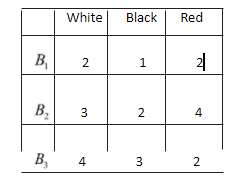Filters
Latest Questions
Mathematics
Baye's theorem
A card from a pack of 52 cards is lost from the remaining cards of the pack, two cards are drawn and are found to be both diamonds. Find the probability of the lost card being a diamond.
Mathematics
Baye's theorem
A bag ‘A’ contains $2$ white and $3$ red balls and bag ‘B’ contains $4$ white and $5$ red balls. One ball is drawn at random from one of the bags and is found to be red. Find the probability that it was drawn from bag ‘B’ was
A) $\dfrac{5}{{14}}$
B) $\dfrac{5}{{16}}$
C) $\dfrac{5}{{18}}$
D) $\dfrac{{25}}{{52}}$
Mathematics
Baye's theorem
The chance that Doctor $A$ will diagnose disease $X$ correctly is 60%. The chance that a patient will die by his treatment after the correct diagnosis is 40% and the chance of death after the wrong diagnosis is 70%. A patient of Doctor $A$ who had disease $X$ died. The probability that his disease was diagnosed correctly is
A. $\dfrac{5}{{13}}$
B. $\dfrac{6}{{13}}$
C. $\dfrac{2}{{13}}$
D. $\dfrac{7}{{13}}$

Mathematics
Baye's theorem
State and prove Bayes’ theorem.
Mathematics
Baye's theorem
In a bolt factory, three machines A, B and C manufacture 25, 35 and 40 percent of the total bolts respectively. Out of the total bolts manufactured by the machines 5, 4 and 2 percent are defective from machine A, B and C respectively. A bolt drawn at random is found to be defective. Find the probability that it was manufactured by (i) Machine A or C (ii) Machine B.

Mathematics
Baye's theorem
A JEE aspirant estimates that she will be successful with an 80 percent chance if she studies 10 hours per day, with a 60 percent chance if she studies 7 hours per day and with 40 percent chance if she studies 4 hours per day. She further believes that she will study 10 hours, 7 hours, and 4 hours per day with probabilities 0.1, 0.2 and 0.7 respectively. Given that she does not achieve success, the chance she studied for 4 hour, is
A.$\dfrac{18}{26}$
B. $\dfrac{19}{26}$
C. $\dfrac{20}{26}$
D. $\dfrac{21}{26}$

Mathematics
Baye's theorem
If ${{E}_{1}}$ and ${{E}_{2}}$ are equally likely, mutually exclusive and exhaustive events and $P\left( A/{{E}_{1}} \right)=0.2$ and $P\left( A/{{E}_{2}} \right)=0.3$ . Then find $P\left( {{E}_{1}}/A \right)$ .
Mathematics
Baye's theorem
Three boxes${B_1}$,${B_2}$,${B_3}$ contain balls with different colours as follows:Mathematics
Baye's theorem
Suppose that 5 men out of 100 and 25 women out of 1000 are good orators. Assuming that there are an equal number of men and women, find the probability of choosing a good orator.

Mathematics
Baye's theorem
A man is known to speak the truth on average $3$ out of $4$ times. He throws a fair dice and reports that its a $6.$ what is the probability it is actually a $6$ .
A. ${\text{ }}\dfrac{3}{5}$
B. ${\text{ }}\dfrac{3}{8}$
C. ${\text{ }}\dfrac{1}{5}$
D. ${\text{ }}\dfrac{3}{4}$
Mathematics
Baye's theorem
There are two boxes $I$ and $II$ . Box $I$ contains 3 red and 6 black balls. Box $I$ contains 5 red and $'n'$ black balls. One of the two boxes, box $I$ and box $II$ is selected at random and a ball is drawn at random. The ball drawn is found to be red. If the probability that this red ball comes out from the box $II$ is $\dfrac{3}{5}$ ​, find the value of $'n'$ .
Mathematics
Baye's theorem
A factory production line is manufacturing bolts using three machines, A, B and C. Of the total output, machine A is responsible for $25\%$, machine B for $35\%$ and machine C for the rest. It is known from previous experience with the machines that $5\%$ of the output from machine A is defective, 4% from machine B and $2\%$ from machine C. A bolt is chosen at random from the production line and found to be defective. What is the probability that it came from machine A.
A.$0.632$
B.$0.362$
C.$0.487$
D.None of these
Prev
1
2
3
4
5